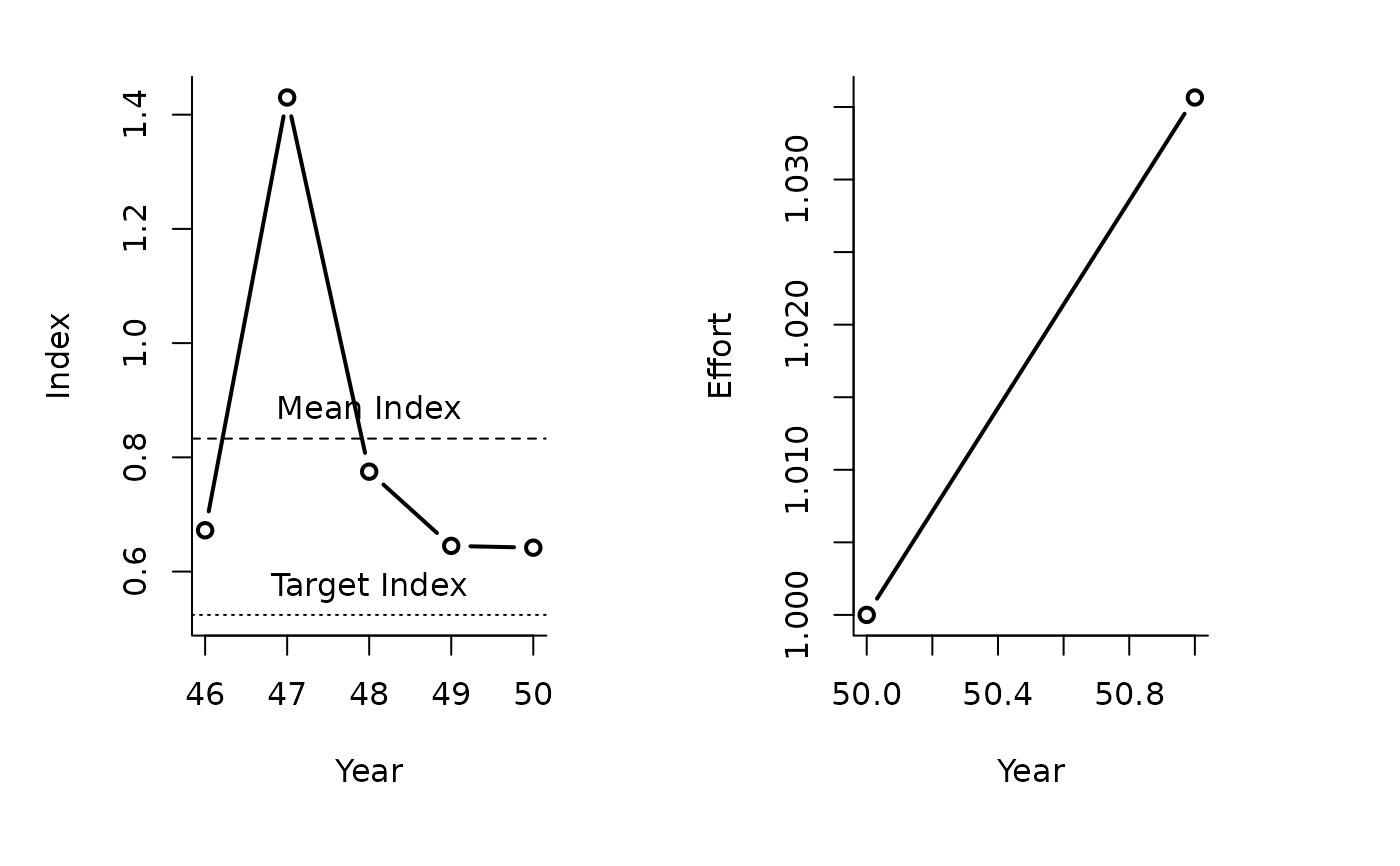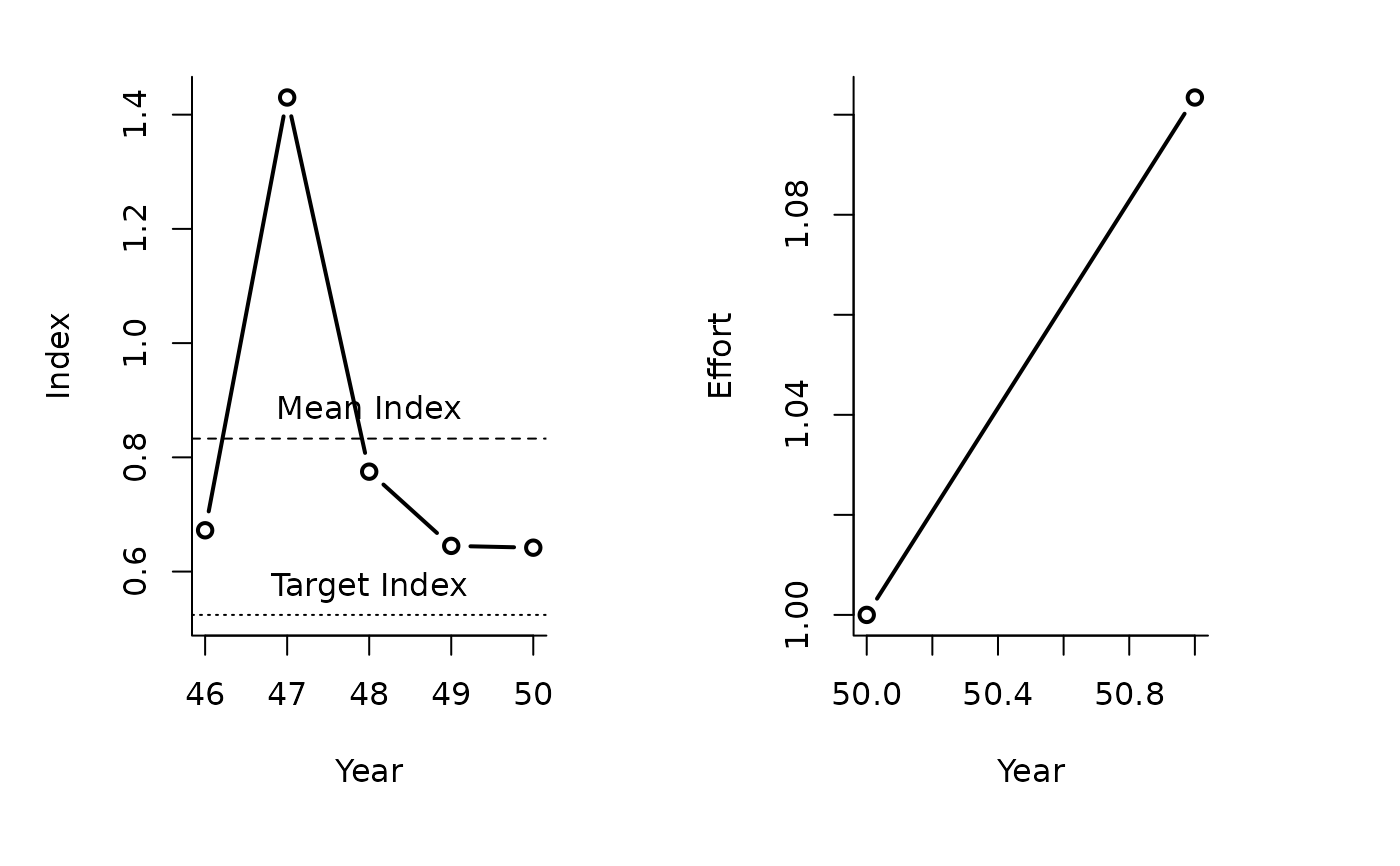An index target MP where the Effort is modified according to current index levels (mean index over last 5 years) relative to a target level.

ITe5(x, Data, reps = 100, plot = FALSE, yrsmth = 5, mc = 0.05)

ITe10(x, Data, reps = 100, plot = FALSE, yrsmth = 5, mc = 0.1)

## Arguments

x

A position in the data object

Data

A data object

reps

The number of stochastic samples of the MP recommendation(s)

plot

Logical. Show the plot?

yrsmth

The number of historical years over which to average the index

mc

The maximum fractional change in the effort among years.

## Value

An object of class Rec-class with the TAE slot(s) populated

## Details

The TAE is calculated as: $$\textrm{TAE}_y = \textrm{TAE}_{y-1} \delta$$ where $$\delta$$ is $$\frac{I} {I_{\textrm{ref}}}$$ averaged over last yrsmth years. $$I_{\textrm{ref}}$$ is the index target (Data@Iref).

The maximum fractional change in TAE is specified in mc.

## Functions

• ITe5: Maximum annual changes are 5 per cent.

• ITe10: Maximum annual changes are 10 per cent.

## Required Data

See Data-class for information on the Data object

ITe5: Ind, Iref, MPeff

ITe10: Ind, Iref, MPeff

## Rendered Equations

See Online Documentation for correctly rendered equations

T. Carruthers

## Examples

ITe5(1, MSEtool::SimulatedData, plot=TRUE)#>   Effort
#> 1.020747
ITe10(1, MSEtool::SimulatedData, plot=TRUE)#>   Effort
#> 1.137569